# Mean + equation - math problems

the arithmetic mean (simply the mean or average) is the sum of a collection of numbers divided by the count of numbers in the collection. The arithmetic mean is the most commonly used and readily understood measure of central tendency in a data set. It is a statistical characteristic of the center of a given data set.

Arithmetic mean is a quantity very sensitive to extreme values, so the median and mode are also used in practice. Mode is the most common value. The median halves the ordered file.

#### Number of problems found: 46

• Three groupsIn the company, employees are divided into three groups. In the first group, which includes 12% of the company's total number of employees, the average salary is CZK 40,000, in the second group CZK 35,000, in the third group CZK 25,000. The average salary
• Beer tapping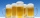When checking compliance with the beer tapping, it was found that 60% of the offered beers were underfilled. The others were fine. Thus, instead of 0.5 l, the volume was 4.4 dcl on average. What was the volume of one average underfilled beer?
• Large familyThe average age of all family members (children, mother, father, grandmother, grandfather) is 29 years. The average age of parents is 40 years, grandparents 66 years and all children are 5 years. How many children are there in this family?
• TroopsThere are two leaders in the tourist group, with an average age of 30 years and several children with an average age of 10 years. The total age of the group is 12 years. How many children are in the group?
• Average monthly salaryA total of 10 teachers work at one small school in Moravia. The monthly salary of each is 21,500 CZK or 21,800 CZK or 22,500 CZK according to their education and age. The average monthly salary for this school's teacher is 21 850 CZK. How many teachers of
• Trees avgThe worker planted 96 trees on Monday, 120 on Tuesday and 61 trees on Wednesday. How many trees did he plant on Thursday if he averaged 105 trees per day?
• Father and sons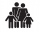Father is 27 and his sons 2 and one year. In how many years will his sons sum up to half his age?
• Five numbers in ratioThere are 5 integers that are in the ratio 1: 2: 3: 4: 5. Their arithmetic mean is 12. Determine the smallest of these numbers.
• AM of three numbersThe number 2010 can be written as the sum of 3 consecutive natural numbers. Determine the arithmetic mean of these numbers.
• The escalator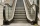I run up the escalator at a constant speed in the direction of the stairs and write down the number of steps A we climbed. Then we turn around and run it at the same constant speed in the opposite direction and write down the number of steps B that I clim
• Between two bus stops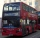Wanda lives between two bus stops at three-eighths of their distance. He started the house today and found that whether he was running to one or the other stop, he would have arrived at the bus stop. The average bus speed is 60 km/h. What is the average s
• Positive integersSeveral positive integers are written on the paper. Michaella only remembered that each number was half the sum of all the other numbers. How many numbers could be written on paper?
• Bonuses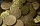Five employees of the company were paid bonuses so that each successor received a 550 USD less than the previous employee. How much did everyone get, if a total of USD 11,000 has paid?
• Centroid - two bodiesA body is composed of a 0.8 m long bar and a sphere with a radius of 0.1m attached so that its center lies on the longitudinal axis of the bar. Both bodies are of the same uniform material. The sphere is twice as heavy as the bar. Find the center of gravi
• MG=7x-15,MG=7x-15, FG=33, x=? Point M is the midpoint of FG. Find unknown x.
• Midpoint 6FM=8a+1, FG=42, a=? Point M is the midpoint of FG. Find unknown a.
• Find midpoint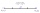FM=5y+13, MG=5-3y, FG=? M is the midpoint of FG. Use the given information to find the missing measure or value.
• Ten cashiers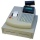Ten cashiers are open at Tesco. Customers wait an average of 15 minutes. How many other cashiers have to open to reduce the waiting time by 4 minutes?
• Average heightThe average height of all pupils is 162 cm. The class teacher's height is 178 cm. The average height of all (teacher and all pupils) is 163 cm. Calculate the number of pupils in the class.
• Average temperatureThe average temperature from Monday to Sunday was 40.5°F and the average temperature from Monday to Saturday was 42.8°F. What was the temperature on Sunday?

Do you have an interesting mathematical word problem that you can't solve it? Submit a math problem, and we can try to solve it.

We will send a solution to your e-mail address. Solved examples are also published here. Please enter the e-mail correctly and check whether you don't have a full mailbox.

Please do not submit problems from current active competitions such as Mathematical Olympiad, correspondence seminars etc...

Looking for help with calculating arithmetic mean? Do you have a linear equation or system of equations and looking for its solution? Or do you have quadratic equation? See also more information on Wikipedia.